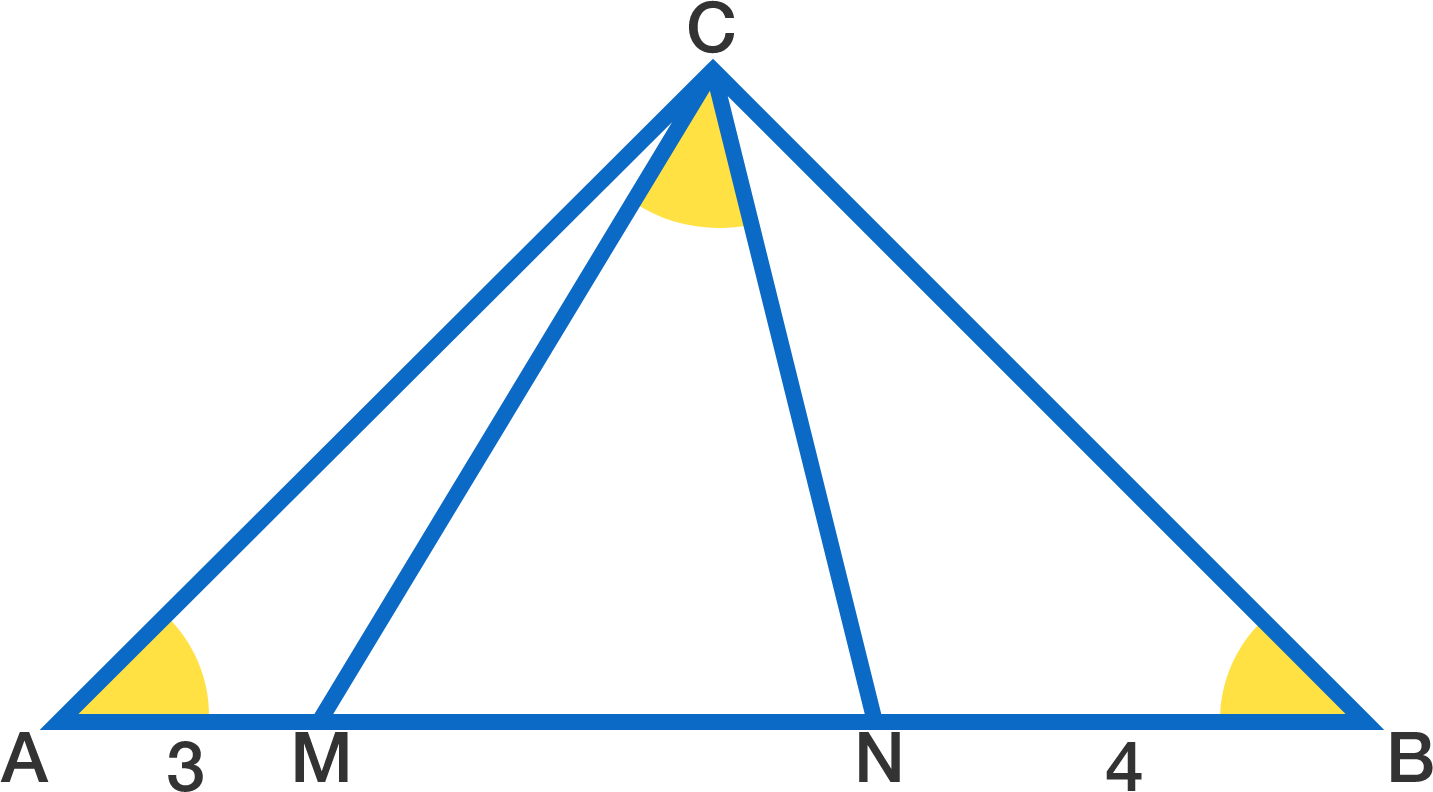# $45°$ is everywhere

Geometry Level 2$M$ and $N$ are points on hypotenuse $AB$ of right triangle $ABC$ such that $AM=3, NB= 4.$

If all three of the yellow angles measure the same, what is the area of $\triangle ABC?$

×